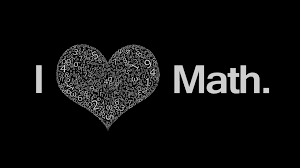## Try, improve, learn & sharE1: -18X2 +15X +3 = 0
30Y2 +16Y +2 = 0
(a) X > Y
(b) X ≤ Y
(c) X < Y
(d) X ≥ Y
(e) Relationship between X and Y cannot be established

2: - 12X2 + 17X +6 = 0
9Y2 +27Y + 20 = 0
(a) X > Y
(b) X ≤ Y
(c) X < Y
(d) X ≥ Y
(e) Relationship between X and Y cannot be established

3: - 6X2 -25X +26 = 0
Y2 -30Y +4 = 0
(a) X > Y
(b) X ≤ Y
(c) X < Y
(d) X ≥ Y
(e) Relationship between X and Y cannot be established

4: - X2 +6X +5 = 0
5Y2 +12Y +4 = 0
(a) X > Y
(b) X ≤ Y
(c) X < Y
(d) X ≥ Y
(e) Relationship between X and Y cannot be established

5: - X = √25
Y = 10 +√16
(a) X > Y
(b) X ≤ Y
(c) X < Y
(d) X ≥ Y
(e) Relationship between X and Y cannot be established

6: - X = √144
Y =7 + √36
(a) X > Y
(b) X ≤ Y
(c) X < Y
(d) X ≥ Y
(e) Relationship between X and Y cannot be established

7: - 676X2 -196X2 = 1920
√256Y3 -8Y3 =27

(a) X > Y
(b) X ≤ Y
(c) X < Y
(d) X ≥ Y
(e) Relationship between X and Y cannot be established

8: - 2X2 +19X +44 =0
2Y2 +3Y -20 = 0
(a) X > Y
(b) X ≤ Y
(c) X < Y
(d) X ≥ Y
(e) Relationship between X and Y cannot be established

9: - 12X +2Y = 3
6X +17Y =9
(a) X > Y
(b) X ≤ Y
(c) X < Y
(d) X ≥ Y
(e) Relationship between X and Y cannot be established

10: - X2 -26X +169 = 0
Y2 -24Y +144 = 0
(a) X > Y
(b) X ≤ Y
(c) X < Y
(d) X ≥ Y
(e) Relationship between X and Y cannot be established

चर्चा व प्रश्नोत्तर हेतु हमें  whatsaap पर सम्पर्क करें: 8853023198, 8299717989
एवं

#### plz Like & Share our efforts with ur friends👈

Math Quiz No.3Reviewed by Ravi kumaR on December 04, 2017 Rating: 5